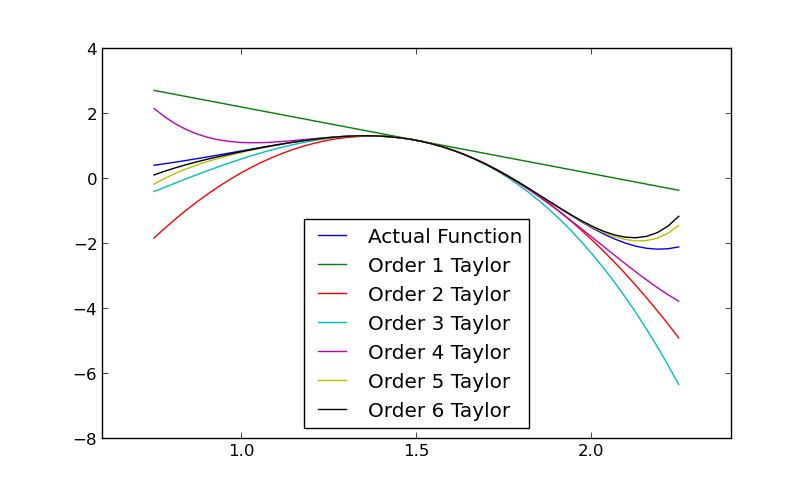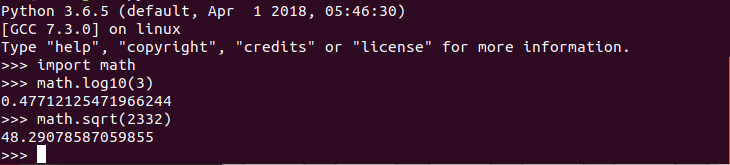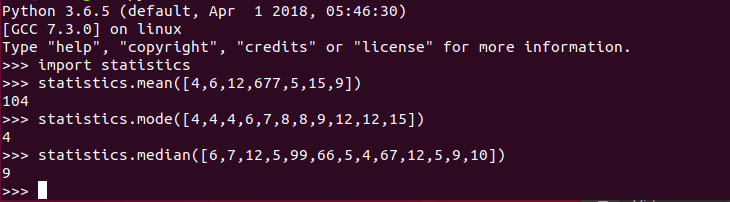# Essential Free Python Maths Tools

Python is a very popular general purpose programming language — with good reason. It’s object oriented, semantically structured, extremely versatile, and well supported. Scientists favour Python because it’s easy to use and learn, offers a good set of built-in features, and is highly extensible. Python’s readability makes it an excellent first programming language.

The Python Standard Library (PSL) is the the standard library that’s distributed with Python. The library comes with, among other things, modules that carry out many mathematical operations.

The math module is one of the core modules in PSL which performs mathematical operations. The module gives access to the underlying C library functions for floating point math.

It offers number-theoretic and representation functions, power and logarithmic functions, trigonometric functions, angular conversion, hyperbolic functions, special functions, and constants. Here’s a quick example:PSL also provides other mathematics functions:

• decimal – provides support for fast correctly-rounded decimal floating point arithmetic.
• fractions – provides support for rational number arithmetic.
• random – implements pseudo-random number generators for various distributions.
• statistics – provides functions for calculating mathematical statistics of numeric data. The statistics module is a relatively late addition; it was added in Python 3.4.
• numbers – numeric abstract base classes.

Here’s the statistics module performing some simple statistical averaging (mean, mode, and median respectively).But PSL is literally the tip of the iceberg. Python has thousands of components available ranging from individual programs and modules to packages and entire application development frameworks. We’ve covered some of these components in other Python articles. Some of the software will be of interest to mathematicians particularly our article on Python Visualization Packages. Software like matplotlib, seaborn, pandas, and Bokeh are some of our go-to libraries.

We want to share some great libraries and tools that are useful for mathematicians working with Python. All of the 8 software receive our strongest recommendation. They expand immensely on the mathematics functionality provided by the Python Standard Library. They are all released under an open source license.Python Mathematics Tools
NumPyCore package for scientific computing with Python
SciPyEcosystem for mathematics, science, and engineering.
statsmodelsStatistical modeling and econometrics
SymPyLibrary for symbolic mathematics
mpmathLibrary for arbitrary-precision floating-point arithmetic
SageMathComputer algebra system
patsyPackage for describing statistical models and to build design matrices
1.William P says: